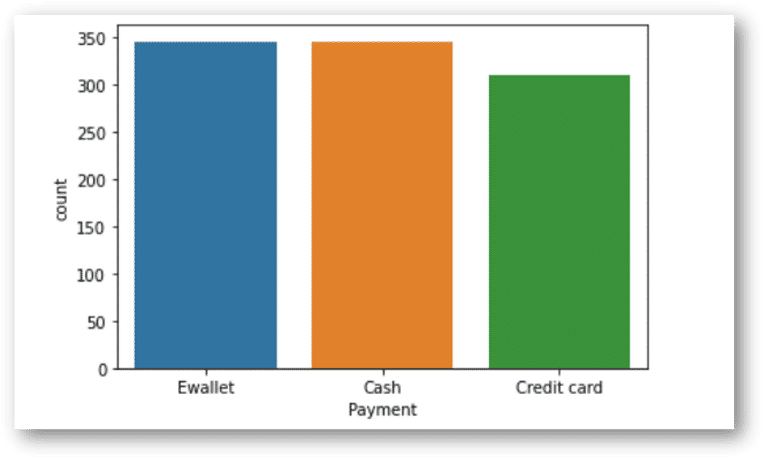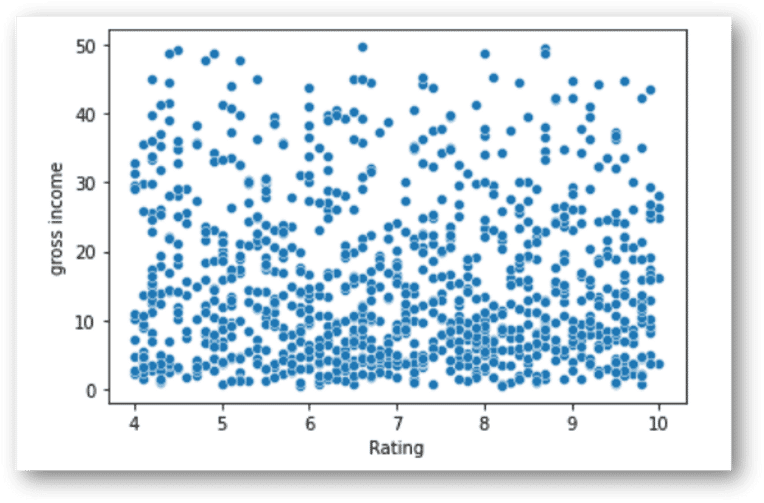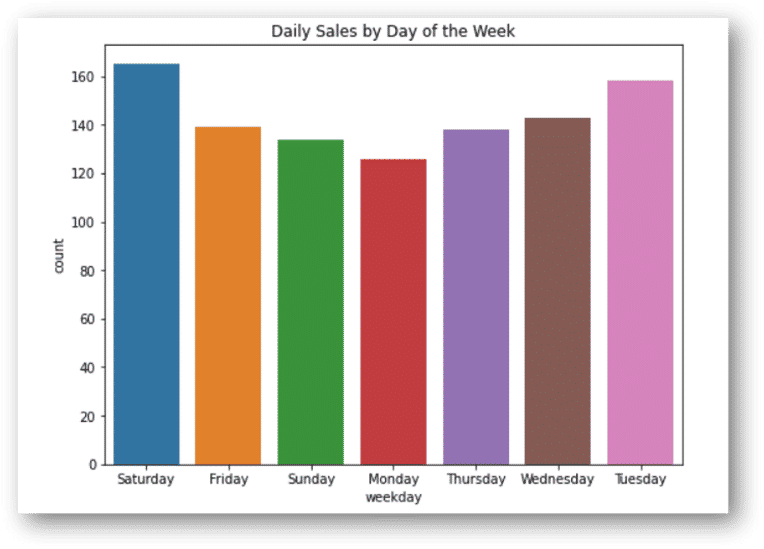# We will consider the supermarket sales data from the Kaggle dataset.Mythili Krishnan

2 years ago | 8 min read

Before venturing on to any data science project it is important to pre-process the data and also to explore the data. Today we will discuss a very basic topic of exploratory data analysis (EDA) using Python and also uncover how simple EDA can be extremely helpful in performing preliminary data analysis.

The approach we will follow today is ask some questions and try to get those answers from the data.

We will consider the supermarket sales data from the Kaggle dataset.

# 1. Familiarize with the dataset and variables:

The dataset consists of historical sales data of a supermarket company from 3 different branches over 3 months from Jan-March 2019. Following table provides a summary of the variables and the variable description.

The dataset consists of data from 3 cities or 3 branches in Myanmar as given below-

a) Branch A (Yangoon)

b) Branch B (Mandalay)

c) Branch C (Naypyitaw)

# 2. Uni-variate analysis

Uni-variate analysis is the analysis involving a single variable (‘uni’) without considering relationships with other variables. This is the stand-alone analysis of a variable/column without considering any casual relationships with other variables. We will see how a simple uni-variate analysis can help to get more insights into the data.

Question 1: What does the customer rating look like and is it skewed?

The lines of code below can be used to answer this:

sns.distplot(df['Rating'])
plt.axvline(x=np.mean(df['Rating']), c='red', ls='--', label='mean')
plt.axvline(x=np.percentile(df['Rating'],25),c='green', ls='--', label = '25th percentile:Q1')
plt.axvline(x=np.percentile(df['Rating'],75),c='orange', ls='--',label = '75th percentile:Q3' )
plt.legend()
Ans: The rating distribution looks uniform and there seems to be no skewness on the left or right side of the distribution. We can plot the mean and the percentiles to show this as well. The red dotted lime is the mean and the green and orange lines indicate the 25th and 75th percentiles respectively.The mean rating is ~7 across products.

Question 2: Is there any difference in aggregate sales across branches?

Next we would like to know whether there is any difference in aggregate sales across the branches.This can be achieved by a count plot as given below:

sns.countplot(df['Branch'])
df['Branch'].value_counts()A 342
B 333
C 328
Name: Branch, dtype: int64
Ans: There is not much difference in sales across the 3 branches of A, B and C. The sales in branch A is a bit higher than the rest of the branches.

Question 3: Which is the most pouplar payment method used by customers?

We can again do this using count plot as given below:

sns.countplot(df['Payment'])Fig 3: Most popular payment method (E wallet): Image by author
Ans: The most popular payment method is in-fact E-wallet and not credit cards.Cash payment is also popular.

# 3. Bi-variate analysis

Let us now consider two (bi) variables together and look at the interaction/relationship between them. This is bi-variate analysis that can help to draw important conclusions about the data.

Let us first consider gross income and try to answer the following questions:

Question 4: Does gross income affect the ratings that the customers provide?

We can use scatter plot and regression plot to answer this question.We can use the following code:

sns.scatterplot(df['Rating'], df['gross income'])

The output will look like this:Fig 4: Scatter plot: Gross income vs Rating (Image by Author)

We can also fit a trend-line to this plot using the regression plot as given below.

Ans: As you can see from the scatter plot and the trend line which is pretty flat that there is no relationship between gross income of a customer and his rating.

Question 5: Which branch is the most profitable?

We can use use the box plot given below for this.

sns.boxplot(x=df['Branch'], y=df['gross income'])
Ans: There is not much difference in gross income by branches at an average level. Branch C has a slightly higher income than A or B.As observed earlier,though branch A has slightly higher sales than the rest,C i.e. Naypyitaw is the most profitable branch in terms of gross income.

Question 6: Is there any relationship between Gender and Gross income?

Similar to the branch analysis earlier,we can use a box plot to answer this question.

sns.boxplot(x=df['Gender'], y=df['gross income'])
Ans: Gross income is similar for both male and female, though female customers spend a bit higher at the 75th percentile.

Question 7: Is there any time trend in gross income?

There are multiple dates per customers, so we have to summarize the data to find the time trend of gross income. We will use ‘date’ as the index for this and for summarization we will use mean value of the variables.

sns.lineplot(x= df.groupby(df.index).mean().index,
y = df.groupby(df.index).mean()['gross income'])
Ans: No particular time trend is observed except for some days when the gross income is pretty high or pretty low. Overall it remains at a certain average level.

Question 8: Which product line generates most income?

cat=df[["Product line", "gross income"]].groupby(['Product line'], as_index=False).sum().sort_values(by='gross income', ascending=False)
plt.figure(figsize=(20,8))sns.barplot(x='Product line', y='gross income', data=cat)
Ans: Gross income is highest in sports and travel.

# 3. Correlation analysis

Correlation is the degree to which a pair of variables is linearly related.

Correlation analysis indicates the positive or negative or zero correlation between the variables. A positive correlation means that the values of the 2 variables increase together and negative correlation means the values of one variable decrease with the other.Zero correlation implies no correlation.

The seaborn heat-map can be used to visualize the correlations among variables.

sns.heatmap(np.round(df.corr(),2), annot=True)

As you can see, unit price is positively correlated to cogs with 63% correlation. Another interesting observation is that ‘Ratings’ hardly has any correlation with any other variables.

As is obvious, Quantity and gross income has very high correlation of 70%.

# 4. Other analysis

Question 9: What is the spending pattern of females and males and in which category do they spend a lot?

For the analysis on spending patterns of males and females, we can create create a dummy variable for Gender and concatenate it with the original data.(You can find the code for this here).

We can also utilize the ‘hue’ option to get this information in one chart.

plt.figure(figsize=(12, 6))
plt.title('Total Monthly transaction by Gender')
sns.countplot(df['Product line'], hue = df.Gender)
Ans: Females spend on 'fashion accessories' the most and for males it is 'Health and beauty'. Females also spend more on 'Sports and travel'.

Question 10: How many products are bought by customers?

Using distribution plot and heat map we can explore the number of products/quantities that most customers buy.

xdata = [1,2,3,4,5,6,7,8,9,10]
plt.figure(figsize = (12,6))
sns.distplot(df['Quantity'])
plt.xticks(xdata)
Ans: Most of the customers buy 10 quantities.

Question 11: Which day of the week has maximum sales?

Let us now explore if there is any particular day of the week when the sales is higher.

plt.figure(figsize=(8, 6))
plt.title('Daily Sales by Day of the Week')
sns.countplot(df['weekday'])Fig 13: Daily sales by day of the week (Image by Author)
Ans: Sales is highest on Saturdays probably because it is the weekend. Interestingly,Tuesdays is a close second.Mondays is the lowest in sales, probably because it is start of the working week.

Question 12: Which hour of the day is the busiest?

We need to extract the hour from the ‘Date’ variable to do this analysis. This can be done in the following way.

df['Time'] = pd.to_datetime(df['Time'])
df['Hour'] = (df['Time']).dt.hour
df['Hour'].unique()

Plotting the data we get the below output:

sns.lineplot(x="Hour", y = 'Quantity',data =df).set_title("Product Sales per Hour")
Ans:Peak is observed in the 14th hour i.e 2 pm of the day. Hence, sales is typically higher in the afternoons.

Question 13: Which product line should the supermarket focus on?

To answer this question, let us look at 2 graphs below:

a) Rating of products: Rating is similar across products and at around 7.

xdata = [0,1,2,3,4,5,6,7,8,9,10]
plt.figure(figsize = (12,6))
sns.barplot(y = df['Product line'], x = df['Rating'])
plt.xticks(xdata)

b) Quantity purchased by product: The mean quantity is lower for ‘fashion accessories’ and ‘food and beverages’

sns.boxenplot(y = 'Product line', x = 'Quantity', data=df )
Ans: Though the rating for ‘fashion accessories’ and ‘food and beverages’ is high but quantity purchased is low. Hence, supply for these products need to be increased.

Question 14: Which city should be chosen for expansion and which products should it focus on?

Let us first answer which city should be chosen for expansion:

plt.figure(figsize=(20,7))
sns.barplot(df['City'],df['gross income'],palette='Set1')
plt.xlabel('City name',fontsize='16')
plt.xticks(fontsize='16')
plt.ylabel('Gross income',fontsize='16')
plt.yticks(fontsize='16')
Ans: It is obvious that Naypyitaw is the most profitable city, hence the expansion plan should be based on this city.

Let us look at the products sold at ‘Naypyitaw’

plt.figure(dpi=125)
sns.countplot(y ='Product line', hue = "City", data = df)
plt.xlabel('Count')
plt.show()
Ans: Fashion accessories and food and beverages are the most sold product in Naypyitaw and these products should be focused on for expansion along with electronic accessories.

### Conclusion:

We used uni-variate, bi-variate and correlation analysis to perform basic EDA on the supermarket sales data.

To summarize below are some of the findings/observations from the data:

1. The customer rating is more or less uniform with the mean rating being around 7 and there is no relationship between gross income and customer ratings.
2. The data consists of 3 cities/branches. Though branch A has slightly higher sales than the rest, C i.e. Naypyitaw is the most profitable branch in terms of gross income.
3. Fashion accessories and food and beverages are the most sold product in Naypyitaw and these products should be focused on along with electronic accessories.
4. The most popular payment method is E-wallet and cash payment is also on the higher side.
5. There is no particular time trend that can be observed in gross income.
6. At an overall level, ‘Sports and Travel’ generates highest gross income.
7. Gross income is similar for both male and female, though female customers spend a bit higher at the 75th percentile. Females spend on ‘fashion accessories’ the most and for males surprisingly it is ‘Health and beauty’. Females also spend more on ‘Sports and travel’ which generates highest income overall.
8. Using the correlation analysis, one interesting observation has emerged that customer ratings is not related to any variable.
9. Most of the customers buy 10 quantities and busiest time of the day is afternoon i.e. around 2 pm which records highest sales. Sales is higher on Tuesdays and Saturdays compared to the rest of the week.
10. Though the rating for ‘fashion accessories’ and ‘food and beverages’ is high but the quantity purchased is low. Hence, supply for these products need to be increased.

All the analysis can be accessed here on Github.

UpvoteCreated by

Mythili KrishnanPost

Upvote

Downvote

Comment

Bookmark

Share

Related Articles